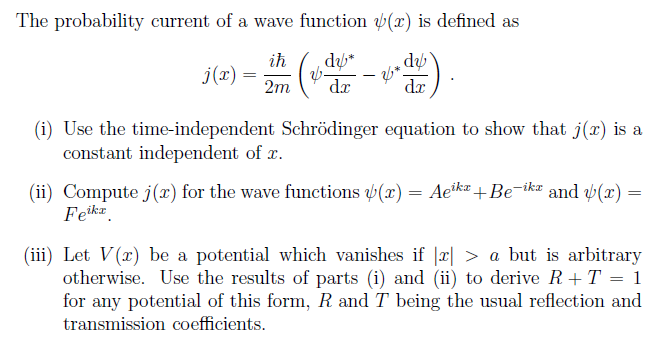# Find j(x). What type of wave this is describing?

##Feb 13, 2018

$j \left(x\right)$ is NOT a wave function! It does not describe a wave at all. In fact, we could choose any arbitrary wave, and it would not change the definition of $j \left(x\right)$.

The probability current, $j \left(x , t\right)$, is the flow of probability through a certain unit area per unit time. We could then predict that $j \left(x\right)$ is a constant w.r.t. position, as the probability density does not vary if we only look at some initial state $j \left(x , 0\right)$.

$\left(i\right)$

Well, if we want to show that $j \left(x\right)$ is a constant w.r.t. $x$, we just need

$\frac{\mathrm{dj} \left(x\right)}{\mathrm{dx}} = 0$.

So... we first find the form of $\frac{\mathrm{dj} \left(x\right)}{\mathrm{dx}}$.

(dj(x))/(dx) = d/(dx)[(iℏ)/(2m)(psi (dpsi^"*")/(dx) - psi^"*" (dpsi)/(dx))]

= (iℏ)/(2m)(psi (d^2psi^"*")/(dx^2) + cancel((dpsi)/(dx) (dpsi^"*")/(dx)) - psi^"*" (d^2psi)/(dx^2) - cancel((dpsi^"*")/(dx)(dpsi)/(dx)))

The time-independent Schrodinger equation is

-ℏ^2/(2m) (d^2psi)/(dx^2) + V(x)psi = Epsi

so we have:

(d^2psi)/(dx^2) = -(2m(E-V(x)))/(ℏ^2)psi

(d^2psi^"*")/(dx^2) = -(2m(E-V(x)))/(ℏ^2)psi^"*"

Therefore:

(dj(x))/(dx) = (iℏ)/(2m)(psi [-(2m(E-V(x)))/(ℏ^2)psi^"*"] - psi^"*" [-(2m(E-V(x)))/(ℏ^2)psi])

= -(iℏ)/(2m)((2m(E-V(x)))/(ℏ^2)psipsi^"*" - (2m(E-V(x)))/(ℏ^2)psi^"*"psi)

Obviously, $\psi$ commutes with ${\psi}^{\text{*}}$, so

color(blue)((dj(x))/(dx)) = -(iℏ)/(2m) cdot (2m(E-V(x)))/(ℏ^2) cdot (psi^"*"psi - psi^"*"psi)

$= \textcolor{b l u e}{0}$

Thus, $j \left(x\right)$ is a constant w.r.t. $x$.

$\left(i i\right)$

We consider the plane wave $\psi \left(x\right) = A {e}^{i k x} + B {e}^{- i k x}$, with $k$ being the usual wave number k = p/ℏ.

It has some probability of reflection or transmission (we do not know what, until we know the properties of $V$ as a function of $x$), and its derivatives are:

$\frac{\mathrm{dp} s i}{\mathrm{dx}} = i k A {e}^{i k x} - i k B {e}^{- i k x}$

(dpsi^"*")/(dx) = -ikA^"*"e^(-ikx) + ikB^"*"e^(ikx)

Therefore, for this $\psi \left(x\right)$:

color(blue)(j_1(x)) = (iℏ)/(2m)[(Ae^(ikx) + Be^(-ikx))(-ikA^"*"e^(-ikx) + ikB^"*"e^(ikx)) - (A^"*"e^(-ikx) + B^"*"e^(ikx))(ikAe^(ikx) - ikBe^(-ikx))]

= (iℏ)/(2m)[-ikA A^"*" + cancel(ikAB^"*"e^(2ikx)) - cancel(ikBA^"*"e^(-2ikx)) + ikBB^"*" - ikA A^"*" + cancel(ikBA^"*"e^(-2ikx)) - cancel(ikAB^"*"e^(2ikx)) + ikBB^"*"]

= (iℏ)/(cancel(2)m)[cancel(2)ik(B B^"*" - A A^"*")]

= color(blue)((ℏk)/m(A A^"*" - B B^"*"))

For $\psi \left(x\right) = F {e}^{i k x}$,

$\frac{\mathrm{dp} s i}{\mathrm{dx}} = i k F {e}^{i k x}$

(dpsi^"*")/(dx) = -ikF^"*"e^(-ikx)

Thus:

color(blue)(j_2(x)) = (iℏ)/(2m)[Fcancel(e^(ikx))(-ikF^"*"cancel(e^(-ikx))) - F^"*"cancel(e^(-ikx))(ikFcancel(e^(ikx)))]

= (iℏ)/(2m)[-ikF F^"*" - ikF F^"*"]

= (iℏ)/(cancel(2)m)[-cancel(2)ikF F^"*"]

= color(blue)((ℏk)/(m)F F^"*")

The terms $A {A}^{\text{*}}$, etc. indicate the probability of the event occurring corresponding to its ${e}^{\pm i k x}$ term. Of course, this means that

• the first plane wave has some finite probability of moving forward (${e}^{i k x}$) or moving backward (${e}^{- i k x}$).
• the second plane wave obviously has a 100% probability of moving forward (e^(ikx) (how else would it move?).

$\left(i i i\right)$

This is a pretty roundabout way of simply saying to place a barrier of arbitrary height in front of the particle of width $2 a$ centered at $x = 0$:

$V \left(x\right) = \left\{\begin{matrix}0 & | x | > a \\ {V}_{0} & - a \le x \le a\end{matrix}\right.$Here we define $k$ on the left of the barrier and $k '$ on the right of the barrier ($k \ne k '$). That is, we define the wave functions for the left and right of the barrier as:

${\psi}_{L} \left(x\right) = A {e}^{i k x} + B {e}^{- i k x}$

${\psi}_{R} \left(x\right) = F {e}^{i k ' x}$

where ${\psi}_{R} \left(x\right)$ necessarily has no $G {e}^{- i k ' x}$ term so that the particle does not double back.

Therefore, what we got in $\left(i i\right)$ is now specified:

j_L(x) = (ℏk)/m(A A^"*" - B B^"*") = "const"

j_R(x) = (ℏk')/mFF^"*" = "const"

From $\left(i\right)$, since $j \left(x\right)$ is a constant w.r.t. $x$ (which we see here) ${j}_{L} \left(x\right) = {j}_{R} \left(x\right) = \text{same const}$.

The reflection coefficient is defined as

$R = \left(B {B}^{\text{*")/(A A^"*}}\right)$

And the transmission coefficient is defined as

$T = \frac{k '}{k} \left(F {F}^{\text{*")/(A A^"*}}\right)$

First, we can say that

overbrace(cancel((ℏ)/m)k(A A^"*" - B B^"*"))^(j_L(x)) = overbrace(cancel((ℏ)/m)k'FF^"*")^(j_R(x))

or that

-(A A^"*" - B B^"*") = B B^"*" - A A^"*" = -(k')/k FF^"*"

Therefore, we use our results to find:

$\textcolor{b l u e}{R + T} = \left(B {B}^{\text{*")/(A A^"*") + (k')/(k)(F F^"*")/(A A^"*}}\right)$

$= \left(B {B}^{\text{*" + A A^"*" - A A^"*")/(A A^"*") + (k')/(k)(F F^"*")/(A A^"*}}\right)$

$= 1 + \left(B {B}^{\text{*" - A A^"*")/(A A^"*") + (k')/(k)(F F^"*")/(A A^"*}}\right)$

$= 1 - \cancel{\frac{k '}{k} \left(F {F}^{\text{*")/(A A^"*") + (k')/(k)(F F^"*")/(A A^"*}}\right)}$

$= \textcolor{b l u e}{1}$

This result should make sense.

Obviously, a particle can only reflect or transmit through a potential barrier. There is nothing else it can physically do, so this total probability should be 100%.